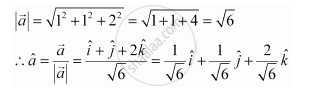Share

# Find the Unit Vector in the Direction of the Vector Veca = Hati + Hatj + 2hatk - CBSE (Science) Class 12 - Mathematics

#### Question

Find the unit vector in the direction of the vector veca = hati + hatj + 2hatk.

#### Solution

The unit vector veca  in the direction of vector  veca = hati + hatj + 2hatk is given by veca = veca/|a|Is there an error in this question or solution?

#### Video TutorialsVIEW ALL 

Solution Find the Unit Vector in the Direction of the Vector Veca = Hati + Hatj + 2hatk Concept: Properties of Vector Addition.
S